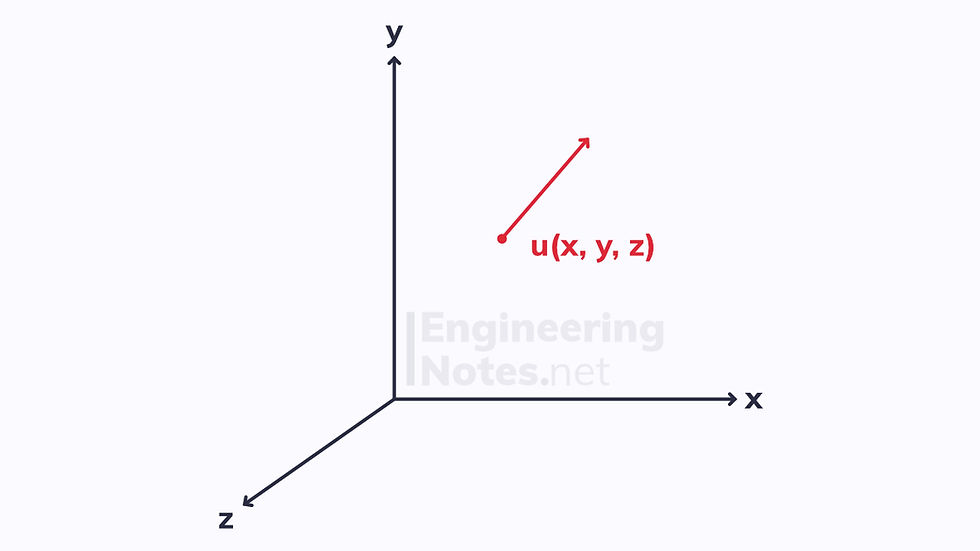top of page

#### Notes by Category University Engineering

Rate these notesNot a fanNot so goodGoodVery goodBrillRate these notes

# Fundamentals of Fluid Mechanics

In fluid mechanics, ‘flow’ is what we call a fluid’s tendency to deform continuously under a shear force. This response to tangential forces and not normal forces is what differentiates fluids from solids:Both the solid and the fluid will deform slightly under the uniform normal force, but then resist any further compression.When a shear (tangential) force is applied to a solid, it deforms slightly in the direction of the force, but then resists further deformation. When a shear force is applied to a fluid, however, it immediately begins to deform, and will continue to do so until the force is removed (it will then eventually come to rest due to the frictional force between the fluid and the container wall).

When acted on by a normal force, solids and fluids behave similarly. When acted on by a shear force, they behave very differently.

As well as differentiating solids and fluids, we must differentiate between liquids and gasses:

• A gas will fill the whole space it is given, a liquid will fill the bottom

• Gasses are far more compressible, as their density is dependent on pressure

## The Continuum Viewpoint

Fluids, like solids, are made up of a vast number of molecules. If we really wanted to, we could therefore investigate the flow and deformation of fluids by looking at the motion of each individual molecule. This is called the molecular viewpoint and is the most fundamental viewpoint – it is used in the pure sciences, but in engineering it is far too complex.

Instead, we make the continuum assumption:

A body consists of infinitely many homogeneous elements, each one significantly smaller than the body itself, but significantly larger than the individual molecules.

We model the elements as homogeneous, as this allows us to define important properties, such as density, temperature etc. at the point of the element.

Assuming that the body is not homogenous on the whole, we can find the average density of the body:If we define an element in the body to have mass δm and volume δV, where the volume tends towards zero, we can work out the density of the individual element:The continuum assumption suggests that as the element gets smaller and smaller, the density does not change.

This assumption is only valid at certain scalesIf δV gets too close to zero, we intrude on the molecular scale where density fluctuates hugely between molecules, if δV is too far from zero, the assumption is vastly inaccurate.

## Field Descriptions

The continuum viewpoint allows us to model properties such as density as a function in space. This is because they are not uniform throughout the body, but they are distributed continuously:Density is a scalar quantity, so the above graph is a ‘scalar field’. If we want to model vector properties, then each plot is a vector from a point, not just a point: it has magnitude and direction as well as location. This is known as a ‘vector field’:• Steady functions do not change with respect to time

• Unsteady functions do change with time

Therefore, we can write functions as:The most common example in fluid mechanics is the velocity field:This is often written in shorthand as:Generally, velocity fields are three dimensional, however sometimes they can be one or two dimensional: